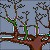## Linear Discriminant Analysis (LDA) Formula

If there are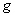groups, the Bayes' rule is minimize the total error of classification by assigning the object to group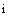which has the highest conditional probability where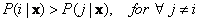. Since we cannot get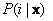(i.e. given the measurement, what is the probability of the class) directly from the measurement and we can obtain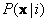(i.e. given the class, we get the measurement and compute the probability for each class), then we use Bayes Theorem: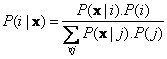Thus, the Bayes' Rule becomes:

Assign the object to groupif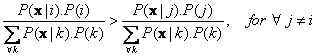The denominators for both sides of inequality are positive and the same, therefore we can cancel them out to become

Assign the object to groupif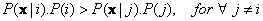If we have many classes and many dimension of measurement which each dimension will have many values, the computation of conditional probabilityrequires a lot of data. It is more practical to assume that the data come from some theoretical distribution. The most widely used assumption is that our data come from Multivariate Normal distribution which formula is given as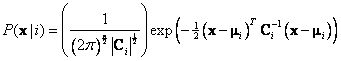Where,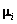is vector mean and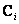is covariance matrix of group i. Inputting the distribution formula into Bayes rule we have:

Assign object with measurement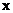to group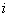ifSince factor of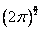are equal for both sides, we can cancel out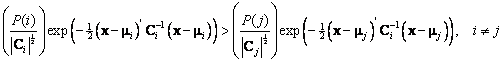Take logarithmic of both sides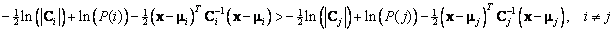Multiply both sides with -2, we need to change the sign of inequality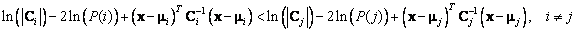Let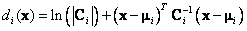we have

Assign object with measurementto groupif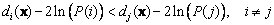If all covariance matrices are equal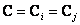, then we can simplify further into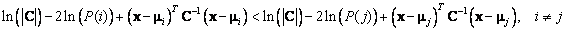We can write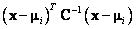into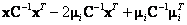. Thus, the inequality becomes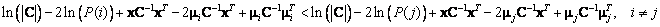We can cancel out the first and third terms (i.e.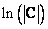and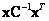) of both sides because they do not affect the grouping decision. Thus, we have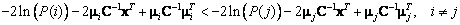We multiply both sides of inequality with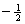(the sign of inequality reverse because we multiply with negative value), we have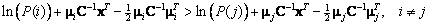Let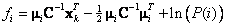, we have

Assign object with measurementto groupif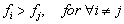That is Linear Discriminant function

Thus, Linear Discriminant Analysis has assumption of Multivariate Normal distribution and all groups have the same covariance matrix.

Preferable reference for this tutorial is

Teknomo, Kardi (2015) Discriminant Analysis Tutorial. http://people.revoledu.com/kardi/ tutorial/LDA/